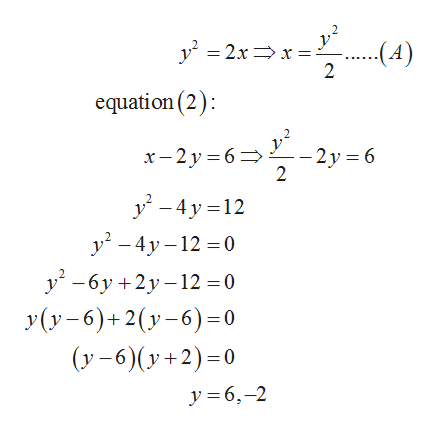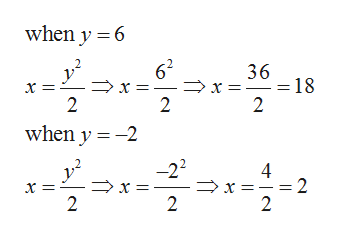# Solve. (If there is no solution, enter NO SOLUTION.)y2 = 2xx − 2y = 6(x, y) =      (smaller x-value)(x, y) =      (larger x-value)

Question
4 views

Solve. (If there is no solution, enter NO SOLUTION.)

 y2 = 2x x − 2y = 6
(x, y)  =

(smaller x-value)
(x, y)  =

(larger x-value)
check_circle

Step 1

It is required to calculate the solution for the given set of equations:

Step 2

Find the value of x from equation (1) and put it in the equation (2)help_outlineImage Transcriptionclosey = 2x=x =: .(4) 2 equation (2): +-3y-6=-y- – 2 y = 6 2 y - 4 y =12 y – 4y – 12 = 0 -6y +2y–12 =0 У(у-6)+2(у-6)-0 (y - 6)(y+2)=0 y = 6,-2 fullscreen
Step 3

Now put the value of y...help_outlineImage Transcriptionclosewhen y = 6 62 > x = 2 v? 36 = 18 when y = -2 -22 > x =-= 2 fullscreen

### Want to see the full answer?

See Solution

#### Want to see this answer and more?

Solutions are written by subject experts who are available 24/7. Questions are typically answered within 1 hour.*

See Solution
*Response times may vary by subject and question.
Tagged in

### Algebra